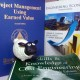# W12_UDS_Emerald Blog Posting Learning Curve

1. Problem Evaluation

We are already in week 13 AACE preparation course; this period is in middle of the course duration. From the beginning until now only blog posting task that have a real and constant target every week. When we make planning time duration (BCWS) 14 weeks ago, we just use our feeling without any formula or technique. This time we want to evaluate our blog posting learning curve to predict our next hour spends. It will be on the track or not (between early and late BCWS) also help us in rebase line our target.Figure 1. Emerald AACE Blog Posting productivity

1. Development of feasible alternatives

Refer to engineering economy book and Humphreys, they are two learning curve methods that we can adopt. Those two methods are:

1. The Unit Linear Learning Curves (ULC)
2. The Cumulative Average Linear Learning Curve (CUMAV)

In this small research, we analyzed our blog posting data in last 13 weeks to predict our next blog posting productivity, it also help us to get some picture of our learning curve in blog posting.

1. Development the outcome for each alternative

This small research methodology is compare two learning curve formula ULC and CUMAV which more appropriate with our behavior blog posting productivity. Regression analysis will use as comparison rating index of both learning curve formulas ULC and CUMAV.

1. Selection of criteria

There are three criteria of the best alternative in this method, there are:

1. The learning rate (s) < 100%, the lower the better
2. Coefficient Determination (R²) the higher the better
3. Learning Curve Exponent (n) < 1, the lower the better.

The chosen alternative should have learning curve fits most of the criteria.

1. Analysis and comparison of the alternative

Because we use group data (each week team productivity) in this research, first we should made lot unit to determine Lot Mid-Point (LMP). LMP formula that we are used is in the below :Figure 2. LMP Equation

After LMP we should determine Average Unit Hour (AUH) of each Lot by dividing the total actual hours spending per lot to the lot size. The equation form default is exponential, it is will be easier and fit with our regression analysis method if we transform it Logarithm Equation below:

Ln (Yx) = Ln (T1) + b x Ln (X)

With definition:

• Ln(Yx) = natural logarithm of each unit hours spend to produce X unit
• Ln (T1) = natural logarithm of the first unit produced
• Ln (X) = natural logarithm of unit X produced
• b = the exponential value associated with the slope

For transform reason we use historical data of blog posting actual hour, the table is below:Table 1. Blog Posting Historical Data

All data being calculated to following table for regression analysis pupose:Table 2. Regression Analysis Calculation

There is no outliers’ data on table above, all data in between LCL and UCL line. In generate regression of each method (ULC and CUMAV) we use excel data analysis and get the summary below:Table 3. ULC and CUMAV Comparison Result

After we get the equation of both method ULC and CUMAV, we can predict of the rest blog posting hour spending, using additional assumption team will produce 7 blog each week so prediction table result is below:Table 4. Blog Posting Hour Projection

1. Alternative selection

From the comparison result table above has shown that The Unit Linear Learning Curves (ULC) Method is considered to be the favorable method to apply, rather than Cumulative Average Linear Learning Curve (CUMAV) Method using Heuristic LMP (Lot Midpoint) since its R² has shown higher rate than the CUMAV. The ULC’s R² value of 0.186 explain that the equation for estimating purpose is best fits with the data being analyzed. While the value of s = 0.72 represented the learning rate of Emerald AACE Team in Blog Writing Project. This number also tells us that Emerald AACE’s skill improvement in doing Blog Writing Project from W1 to W13 have been increased by 28%. The n value, indicate that Emerald AACE Team underspending in this project by (-0.28) and (-0.02) worth.

1. Performance monitoring & Post Evaluation Result

Using appropriate Learning curve method of our team (ULC) can give us a projection of our rest blog writing project especially when we want to rebase line our BACS. It is projection can guide us to plot our new BACS and maintain our CPI on the track. We can do the same evaluation for the other project in couple of weeks, when it already enough data, because the other project target not start in the beginning of preparation course.

References

1. Sullivan, G. W., Wicks, M. E., & Koelling, C. P.(2014). Engineering economy 16th Edition. Chapter 3 – Cost Estimation Techniques., pp.110-113. Prentice Hall.
2. Humphreys, G. (2014). Project Management Using Earned Value (Third Edition). Chapter 22 – Learning Curves., pp.435-441.Humphreys & Assoc.
3. Planning Planet. (2017). Acquiring Man Power For The Project. Retrieved from http://www.planningplanet.com/guild/gpccar/acquiring-manpower-for-the-project
Posted on

## 1 thought on “W12_UDS_Emerald Blog Posting Learning Curve”

1.Dr Paul D Giammalvo says:

Excellent case study Pak Dhanu!!!

Seeing how you are experimenting with learning curves, you may want to challenge yourself to creating a TIME:COST Optimization case study?